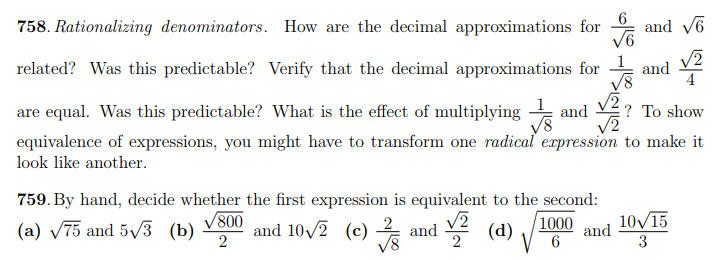Bedford High School

Reflections & 小心

9/29/18

Equivalence is something students need practice with in all grades.  The MCAS 2.0 problems related to this topic have been difficult for our students in middle school .   By the time they get to 11th and 12th grades there still is confusion aboutand.  Students assume that both equations are equivalent. I know this is a fine point and beyond the math standards for 10th grade.

Here are some MCAS 2018 “equivalence” examples from this year’s MCAS.

5th Grade:   Our Lane School students on #5 scored 87% correct and on #4 scored 56% correct, a full 12 points above the state average.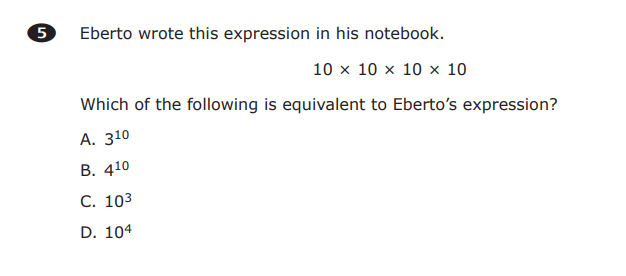6th Grade:  67% of our students answered this one correctly, which was 7% above the state average.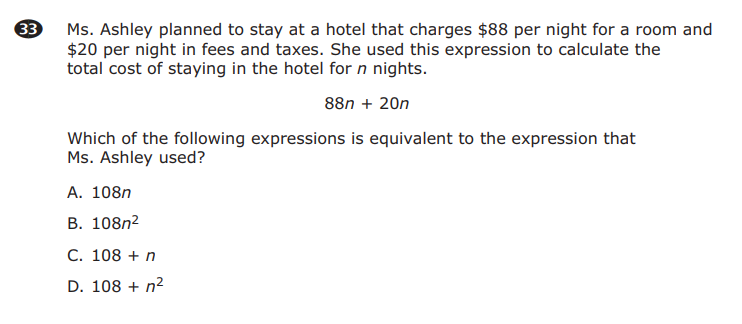6th Grade:  28% correct and we were below the state average by 6 points.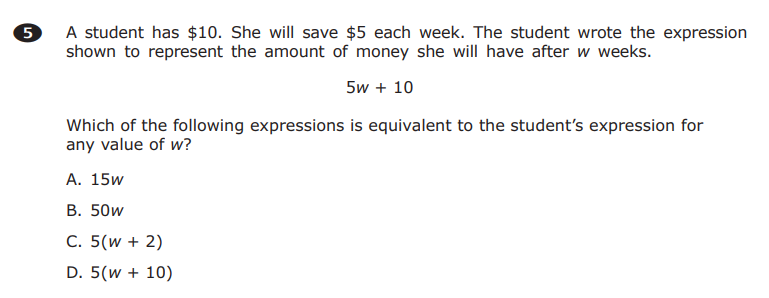7th Grade:  54% correct on this one, 6 points above the state average.  Notice MCAS isn’t concerned with the answer, but an equivalent expression.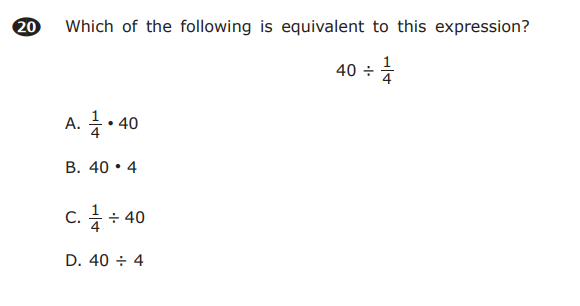7th Grade:  31% of our students got this one correct.  The MCAS is stepping up its game for sure.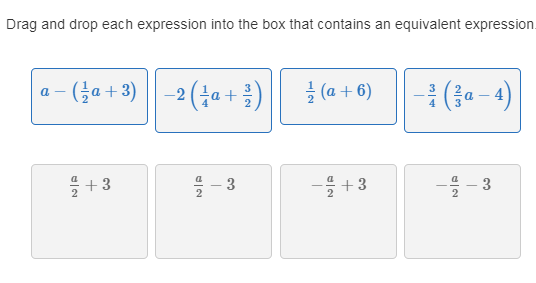8th Grade: 73% of our students got this one correct.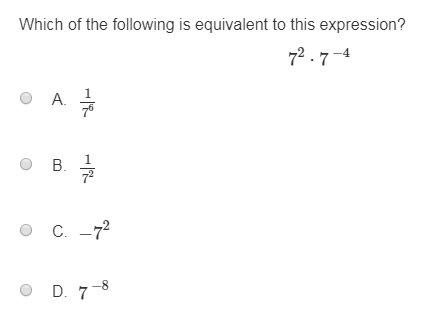10th Grade: 81% correct for BHS students vs. 63% correct for the state.10th Grade:  85% correct vs. 70% for the state.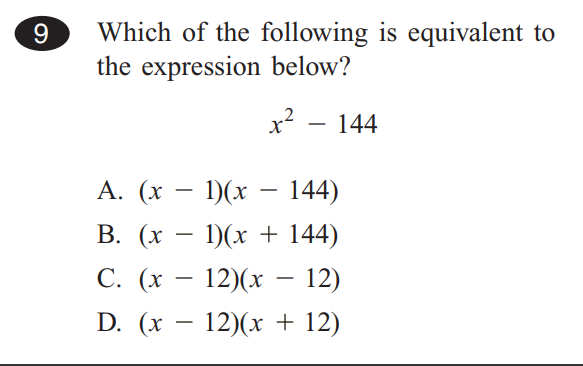I love the resources at Philips Exeter.